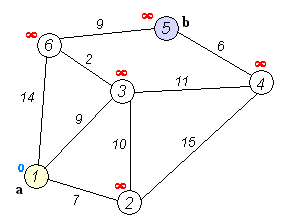# Dijkstra's algorithm

Dijkstra's algorithm is an algorithm to find the shortest path in a weighted graph, which may represent for example, road networks.Dijkstra's algorithm to find the shortest path between a and b.

Breadth-first search will find you the path with the fewest segments. What if you want the fastest path instead? You can do that fastest with a different algorithm called Dijkstra's algorithm.

## Working with Dijkstra's algorithm​

Let's say we have the following graph.Each segment has a travel time in minutes. You'll use Dijkstra's algorithm to go from start to finish in the shortest possible time.

If you ran breath-first search on this graph, you'd get this shortest path.But that path takes 8 minutes. Let's see if you can find a path that takes less time! There are four steps to Dijkstra's algorithm:

1. Find the "cheapest" node. The node you can get to in least amount of time.
2. Update the cost of the neighbors of this node.
3. Repeat until you've done this for every node in the graph.
4. Calculate the final path.

Step 1: Find the cheapest node. You're standing at the start, wondering if you should go to node A or node B. How long does it take to get to each node?It takes 7 minutes to get to node A and 2 minutes to get to node B. The rest of the nodes, you don't know yet.

Because you don't know how long it takes to get to finish yet, you put down infinity. Node B is the closest node... it's 2 minutes away.Step 2: Calculate how long it takes to get to all of node B's neighbors by following an edge from B.You just found a shorter path to node A! It used to take 7 minutes to get to node A.

But if you go through node B, there's a path that only takes 5 minutes.

When you find a shorter path for a neighbor of B, update its cost. In this case, you found

• A shorter path to A (down from 7 minutes to 5 minutes)
• A shorter path to the finish (down from infinity to 7 minutes)

Step 3: Repeat!

At the end the final path should be:Breadth-first search wouldn't have found this as the shortest path, because it has three segments. And there's a way to get from the start to the finish in two segments.## Terminology​

When you work with Dijkstra's algorithm, each edge in the graph has a number associated with it and it's called weights.

A graph with weights is called a weighted graph. A graph without weights is called unweighted graph.

To calculate the shortest path in an unweighted graph, use breath-first search. To calculate the shortest path in a weighted graph, use Dijstra's algorithm.

Graph can also have cycles.

It means you can start at a node, travel around, and end up at the same node. Suppose you're trying to find the shortest path in this graph that has a cycle.

Would it make sense to follow the cycle? Well, you can use the path that avoids the cycle.

Or you can follow the cycle.

You end up at node A either way, but the cycle adds more weigh. You could even follow the cycle twice if you wanted.

But every time you follow the cycle, you're just adding 8 to the total weight. So following the cycle will never give you the shortest path.

An undirected graph means that both nodes point each other. That's a cycle!

Dijkstra's algorithm only works on graphs with no cycles, or on graphs with a positive weight cycle.

## Negative-weight edges​

You can't use Dijkstra's algorithm if you have negative-weight edges.

Negative-weight edges break the algorithm.

Since Dijkstra follows a Greedy Approach, once a node is marked as visited it cannot be reconsidered even if there is another path with less cost or distance. This issue arises only if there exists a negative weight or edge in the graph.

If you want to find the shortest path in a graph that has negative-weight edges, there's an algorithm for that! It's called the Bellman-Ford algorithm.

## Implementation​

Let's see the implemention of the Dijkstra's algorithm:

Graph for the example:Code:

``// Graph initialisationconst graph = {};// Weight representationgraph["start"] = {};graph["start"]["a"] = 6;graph["start"]["b"] = 2;graph["a"] = {};graph["a"]["fin"] = 1;graph["b"] = {};graph["b"]["a"] = 3;graph["b"]["fin"] = 5;graph["fin"] = {};// Cost for each nodeconst infinity = Number.POSITIVE_INFINITY;const costs = {};costs["a"] = 6;costs["b"] = 2;costs["fin"] = infinity;// Parentsconst parents = {};parents["a"] = "start";parents["b"] = "start";parents["fin"] = null;const processed = [];function findLowestCostNode(costs) {  let lowestCost = infinity;  let lowestCostNode = null;  for (let node in costs) {    const cost = costs[node];    if (cost < lowestCost && !processed.includes(node)) {      lowestCost = cost;      lowestCostNode = node;    }  }  return lowestCostNode;}function dijkstras(costs) {  let node = findLowestCostNode(costs);  while (node !== null) {    const cost = costs[node];    const neighbors = graph[node];    for (let n in neighbors) {      const newCost = cost + neighbors[n];      if (costs[n] > newCost) {        costs[n] = newCost;        parents[n] = node;      }    }    processed.push(node);    node = findLowestCostNode(costs);  }}dijkstras(costs);``

## Recap​

• Breadth-first search is used to calculate the shortest path for an unweighted graph.
• Dijkstra's algorithm is used to calculate the shortest path for a weighted graph.
• dijkstra's algorithm works when all the weights are positive.
• If you have negative weights, use the Bellman-Ford algorithm.